# 基本操作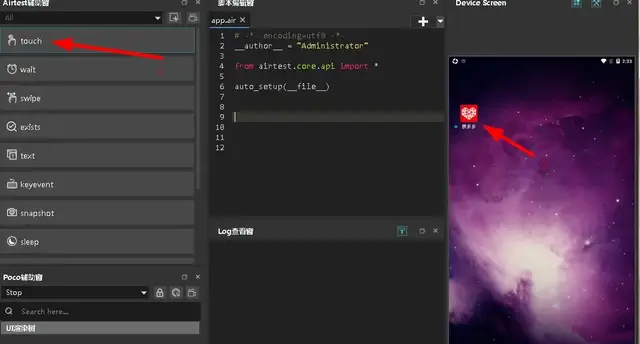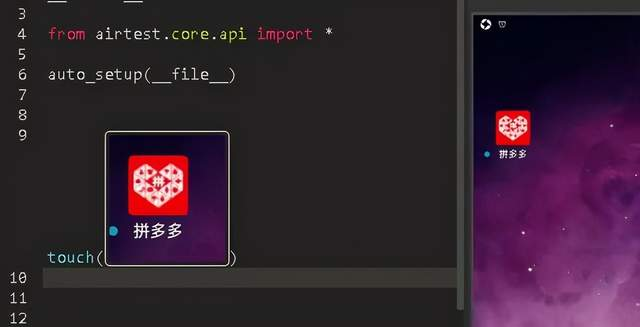# 1.点击目标APP并等待界面加载完毕# 2).根据包名打开

``````dev=device()  # 先获取到当前设备对象,即是Android对象
print(dev.list_app())  # 平台接口列表
start_app('com.tencent.weishi')#利用包名打开微视``````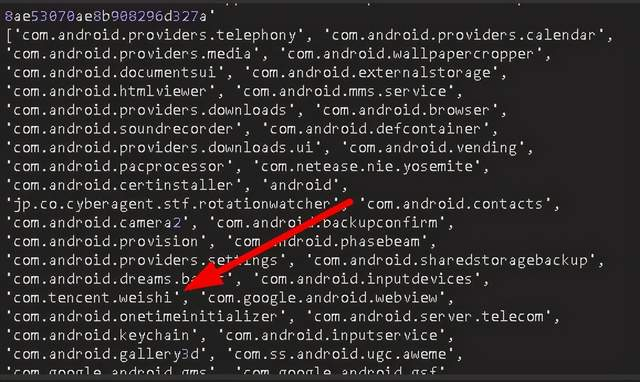# 2.点赞评论关闭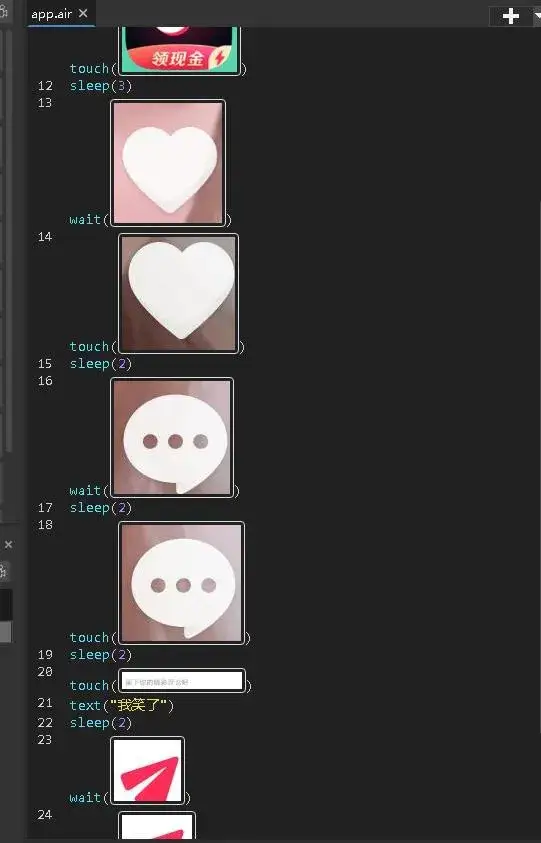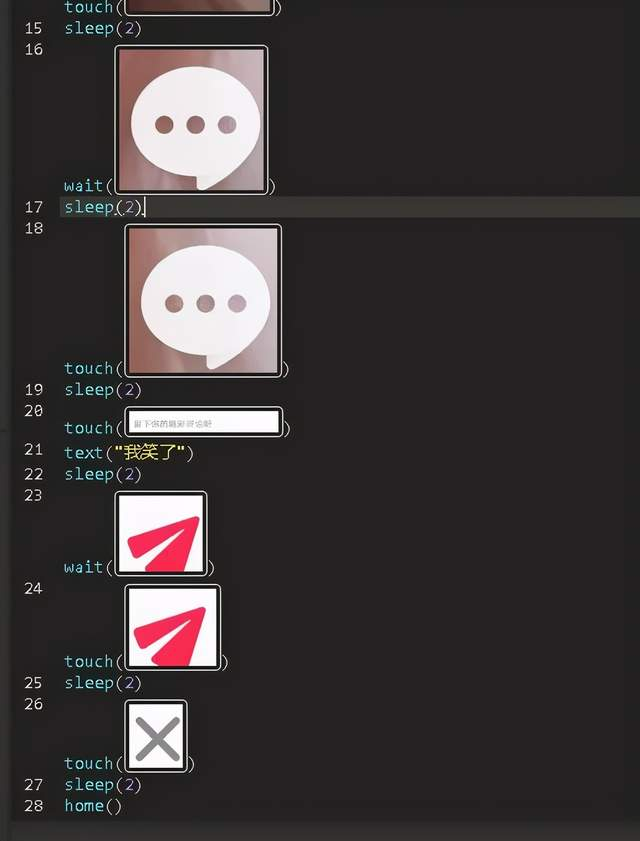# 3.截屏加滑动屏幕

``snapshot(filename=r'C:\Users\Administrator\Desktop\test.jpg',msg='截图成功')``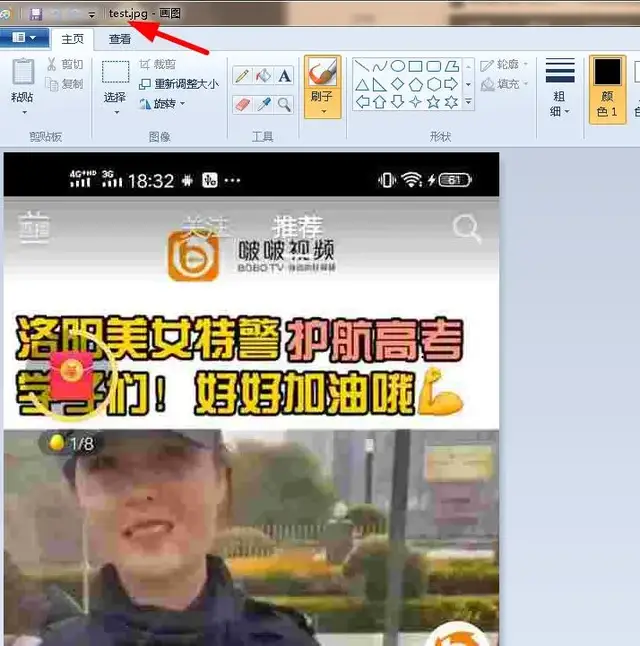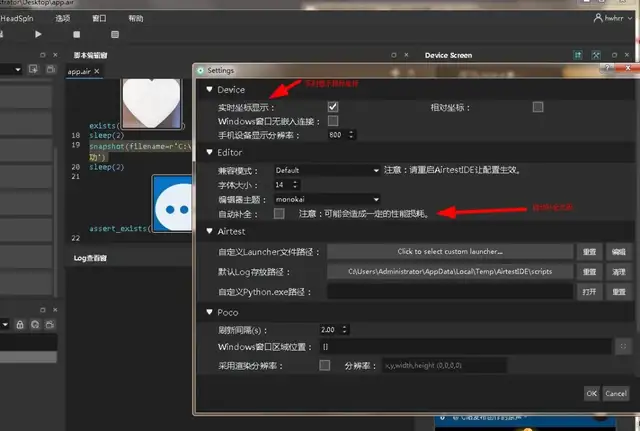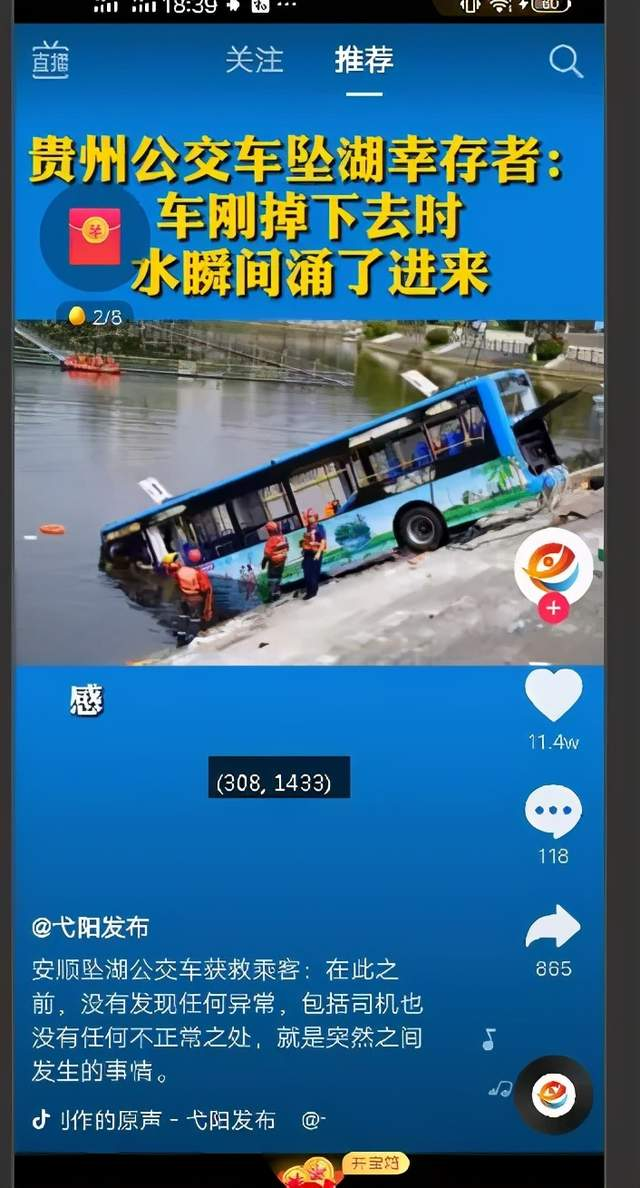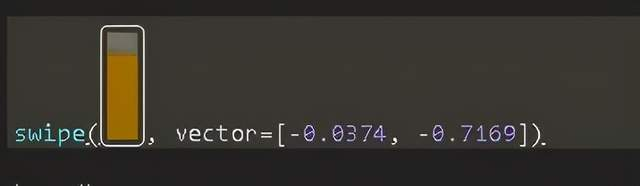# 4.不断滑动屏幕

``````import random

wait(Template(r"tpl1594203055954.png", record_pos=(0.356, 0.562), resolution=(1080, 2340)))
sleep(1)

touch(Template(r"tpl1594203066034.png", record_pos=(0.354, 0.564), resolution=(1080, 2340)))
sleep(2)
exists(Template(r"tpl1594203382253.png", record_pos=(0.415, 0.139), resolution=(1080, 2340)))
sleep(2)
sleep(2)
assert_exists(Template(r"tpl1594203920118.png", record_pos=(0.411, 0.33), resolution=(1080, 2340)), "请填写测试点")

while True:#死循环，不断滑动短视频
aa=random.randint(1,5)
swipe((700,1950),(700,300))
sleep(aa)``````